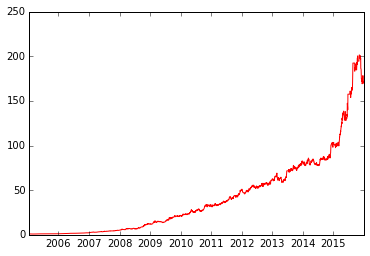# HMM在股票市场的简单应用举一个日常生活中的例子，我们希望根据当前天气的情况来预测未来天气情况。一种办法就是假设这个模型的每个状态都只依赖于前一个的状态，即马尔科夫假设，这个假设可以极大简化这个问题。当然，这个例子也是有些不合实际的。但是，这样一个简化的系统可以有利于我们的分析，所以我们通常接受这样的假设，因为我们知道这样的系统能让我们获得一些有用的信息，尽管不是十分准确的。上面的图显示了天气进行转移的模型。

1. 状态：晴天、阴天和下雨。
2. 初始向量：定义系统在时间为0的时候的状态的概率。
3. 状态转移矩阵：每种天气转换的概率。所有的能被这样描述的系统都是一个马尔科夫过程

1. 知道骰子有几种（隐含状态数量），每种骰子是什么（转换概率），根据掷骰子掷出的结果（可见状态链），我想知道每次掷出来的都是哪种骰子（隐含状态链）。
2. 还是知道骰子有几种（隐含状态数量），每种骰子是什么（转换概率），根据掷骰子掷出的结果（可见状态链），我想知道掷出这个结果的概率。
3. 知道骰子有几种（隐含状态数量），不知道每种骰子是什么（转换概率），观测到很多次掷骰子的结果（可见状态链），我想反推出每种骰子是什么（转换概率）。
如果要解决上面股市中的问题，我们就需要解决问题1和问题3，下面我们就看看如何实现。

HMM在股票市场中的应用

In :
```from hmmlearn.hmm import GaussianHMM
import numpy as np
from matplotlib import cm, pyplot as plt
import matplotlib.dates as dates
import pandas as pd
import datetime
```

In :
```beginDate = '2005-01-01'
endDate = '2015-12-31'
n = 6 #6个隐藏状态
data = get_price('CSI300.INDX',start_date=beginDate, end_date=endDate,frequency='1d')
data[0:9]
```
Out:
MDEntryDate
2005-01-04 982.79 994.77 980.66 20050105 994.77 4431976960 7412869
2005-01-05 992.56 997.32 979.88 20050106 981.58 4529207808 7119109
2005-01-06 983.17 993.79 980.33 20050107 993.33 3921015040 6288028
2005-01-07 983.96 995.71 979.81 20050110 983.05 4737468928 7298694
2005-01-10 993.88 993.96 979.79 20050111 983.76 3762931968 5791697
2005-01-11 997.14 999.55 991.09 20050112 994.19 3704076032 5849079
2005-01-12 996.75 996.98 989.26 20050113 996.65 3093298944 5014525
2005-01-13 996.88 999.47 992.70 20050114 996.08 3842172928 6044065
2005-01-14 988.31 1006.46 987.23 20050117 996.62 4162920960 7297842

In :
```volume = data['TotalVolumeTraded']
close = data['ClosingPx']
```

In :
```logDel = np.log(np.array(data['HighPx'])) - np.log(np.array(data['LowPx']))
logDel
```
Out:
```array([ 0.01428574,  0.01764157,  0.01363667, ...,  0.01380317,
0.01051247,  0.01207808])```

In :
```logRet_1 = np.array(np.diff(np.log(close)))#这个作为后面计算收益使用
logRet_5 = np.log(np.array(close[5:])) - np.log(np.array(close[:-5]))
logRet_5
```
Out:
```array([ 0.01449572,  0.00421252,  0.01384836, ..., -0.03007529,
-0.02652464, -0.02603115])```

In :
```logVol_5 = np.log(np.array(volume[5:])) - np.log(np.array(volume[:-5]))
logVol_5
```
Out:
```array([-0.23693333, -0.35044388, -0.03957071, ..., -0.57079226,
-0.67285963, -0.36793342])```

In :
```logDel = logDel[5:]
logRet_1 = logRet_1[4:]
close = close[5:]
Date = pd.to_datetime(data.index[5:])
```

In :
```A = np.column_stack([logDel,logRet_5,logVol_5])
A
```
Out:
```array([[ 0.00849983,  0.01449572, -0.23693333],
[ 0.00777352,  0.00421252, -0.35044388],
[ 0.00679663,  0.01384836, -0.03957071],
...,
[ 0.01380317, -0.03007529, -0.57079226],
[ 0.01051247, -0.02652464, -0.67285963],
[ 0.01207808, -0.02603115, -0.36793342]])```

In :
```model = GaussianHMM(n_components= n, covariance_type="full", n_iter=2000).fit([A])
hidden_states = model.predict(A)
hidden_states
```
Out:
`array([0, 0, 0, ..., 3, 3, 3])`

spherical：是指在每个马尔可夫隐含状态下，可观察态向量的所有特性分量使用相同的方差值。对应协方差矩阵的非对角为0，对角值相等，即球面特性。这是最简单的高斯分布PDF。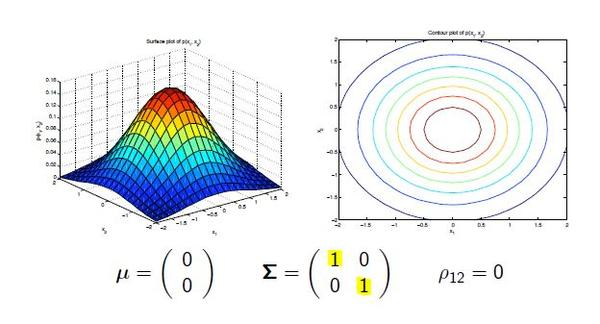diag：是指在每个马尔可夫隐含状态下，可观察态向量使用对角协方差矩阵。对应协方差矩阵非对角为0，对角值不相等。diag是hmmlearn里面的默认类型。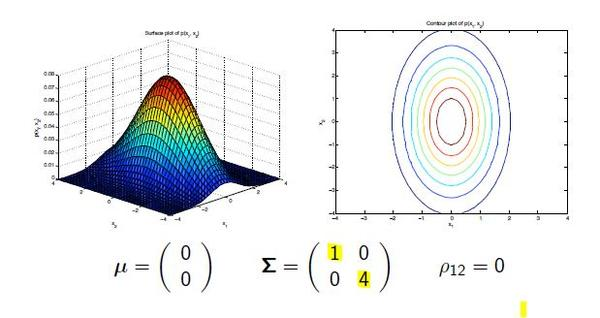full：是指在每个马尔可夫隐含状态下，可观察态向量使用完全协方差矩阵。对应的协方差矩阵里面的元素都是不为零。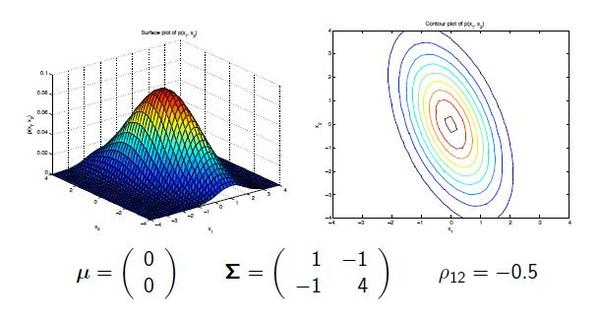tied：是指所有的马尔可夫隐含状态使用相同的完全协方差矩阵。

In :
```plt.figure(figsize=(25, 18))
for i in range(model.n_components):
pos = (hidden_states==i)
plt.plot_date(Date[pos],close[pos],'o',label='hidden state %d'%i,lw=2)
plt.legend(loc="left")
```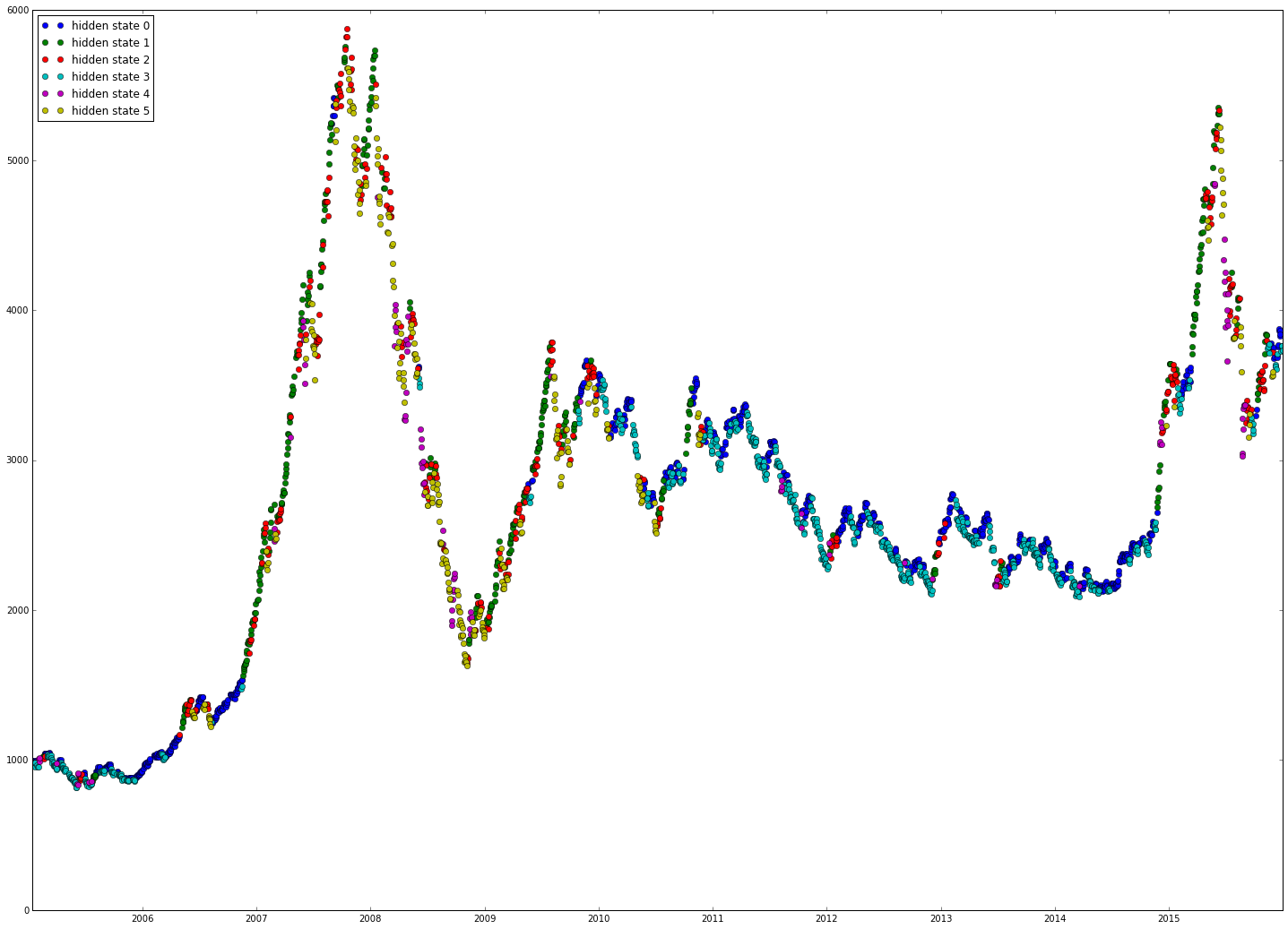In :
```res = pd.DataFrame({'Date':Date,'logRet_1':logRet_1,'state':hidden_states}).set_index('Date')
plt.figure(figsize=(25, 18))
for i in range(model.n_components):
pos = (hidden_states==i)
pos = np.append(0,pos[:-1])#第二天进行买入操作
df = res.logRet_1
res['state_ret%s'%i] = df.multiply(pos)
plt.plot_date(Date,np.exp(res['state_ret%s'%i].cumsum()),'-',label='hidden state %d'%i)
plt.legend(loc="left")
```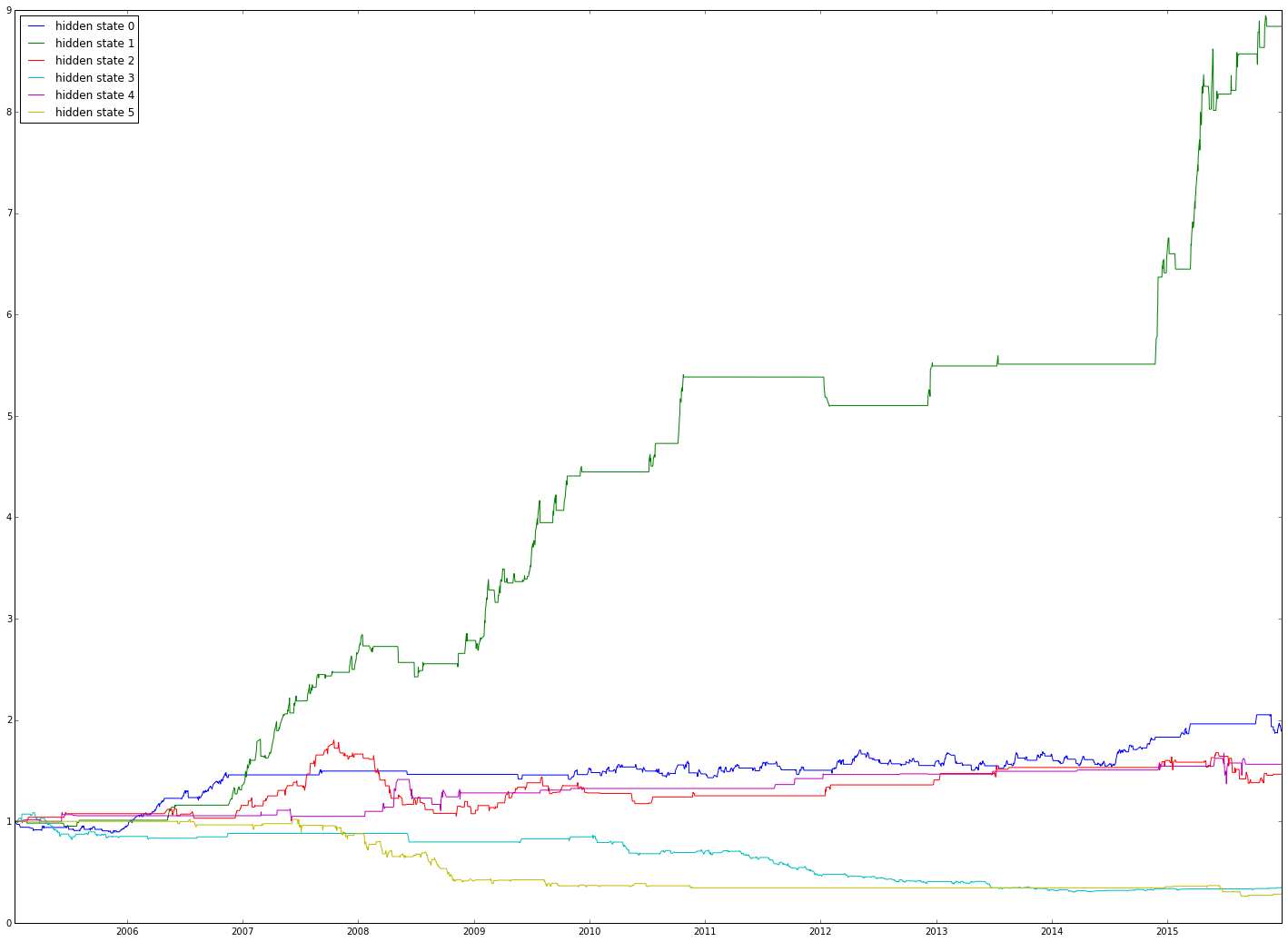In :
```long = (hidden_states==0) + (hidden_states == 1) #做多
short = (hidden_states==3) + (hidden_states == 5)  #做空
long = np.append(0,long[:-1]) #第二天才能操作
short = np.append(0,short[:-1]) #第二天才能操作
```

In :
```res['ret'] =  df.multiply(long) - df.multiply(short)
plt.plot_date(Date,np.exp(res['ret'].cumsum()),'r-')
```
Out:
`[<matplotlib.lines.Line2D at 0x7fb1b67bec50>]`